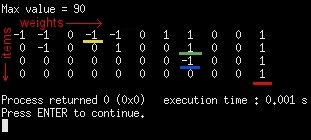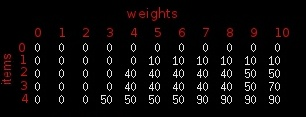# Knapsack Problem Dynamic Programming Algorithm

The Knapsack problem is probably one of the most interesting and most popular in computer science, especially when we talk about dynamic programming.Here’s the description: Given a set of items, each with a weight and a value, determine which items you should pick to maximize the value while keeping the overall weight smaller than the limit of your knapsack (i.e., a backpack).

For example, suppose you are a thief and you invaded a house. Inside you found the following items:

• A vase that weights 3 pounds and is worth 50 dollars.
• A silver nugget that weights 6 pounds and is worth 30 dollars.
• A painting that weights 4 pounds and is worth 40 dollars.
• A mirror that weights 5 pounds and is worth 10 dollars.

Which items should you pick?

Since this is a small problem set it’s not difficult to see the answer is the vase and the painting, for a total value of \$90, but if there were more items computing the answer wouldn’t be so easy.

A brute force approach (i.e., testing all item combinations and keeping the one with the highest value) would take 2^n, where n is the number of items. Once n grows slightly, this approach becomes unfeasible.

What’s a better solution?

We can break the problem into smaller sub-problems (which is called optimal sub-structure in computer science) and solve it recursively (i.e., divide and conquer).

That is, instead of thinking with all the items at the same time, we think about having only one item and a certain size available in the knapsack. With this smaller sub-problem you’ll basically need to decide between two things: to take the item (in which case you get the value of the item but lose capacity in proportion to its weight) or to not take the item (in which case you don’t get any value but don’t lose any weight either). Once you solve this sub-problem you just need to call another recursion, adjusting two things: the item you are working with and the weight you still have available.

One problem that will arise is the re-computation of sub-problems over and over again (which is called overlapping sub-problems). That is because the sub-problems are not independent. When you have this scenario (i.e., optimal sub-structure and overlapping sub-problems) you know what you can use the dynamic programming technique, which basically involved storing the solutions to each sub-problem, so that you just need to compute them once.

Enough talk though, let’s see some code.

### Top-Down Dynamic Programming Solution

Top-down dynamic programming means that we’ll use an intuitive and recursive algorithm to solve the problem, but instead of simply returning the computer values from our function we’ll first store it in an auxiliary table. Then every time we call the recursion we first check the table to see if the solution was computed already. If it was we use it, else we compute and store it for future use.

The algorithm below does exactly that. The parameters of function knapsack are:

int index = index of the item you need to decide to take or not (we start with the last element of the array and we work toward the first)
int size = size still available at the backpack
int weights[] = array with the weights of all items
int values[] = array with the values of all items

``````#include <stdio.h>
#define max(a,b) (a > b ? a : b)

int matrix = {0};

int knapsack(int index, int size, int weights[],int values[]){
int take,dontTake;

take = dontTake = 0;

if (matrix[index][size]!=0)
return matrix[index][size];

if (index==0){
if (weights<=size){
matrix[index][size] = values;
return values;
}
else{
matrix[index][size] = 0;
return 0;
}
}

if (weights[index]<=size)
take = values[index] + knapsack(index-1, size-weights[index], weights, values);

dontTake = knapsack(index-1, size, weights, values);

matrix[index][size] = max (take, dontTake);

return matrix[index][size];

}

int main(){
int nItems = 4;
int knapsackSize = 10;
int weights = {5,4,6,3};
int values = {10,40,30,50};

printf("Max value = %dn",knapsack(nItems-1,knapsackSize,weights,values));

return 0;
}
``````

### Storing the Picked Items

As you can see from the code above it returns the max value you can take, but it doesn’t store what items you need to pick in that optimal solution. To achieve that we need to add another auxiliary table which will keep track, for each combination of index and size available, whether you picked the item or didn’t (i.e., whether the take variable was bigger than the dontTake one).

Once you run the program the table with the picks will look like this:We need to start with the value in the bottom-right (underlined in red). That is the decision of the last item (i.e., the first one we considered) with the backpack completely empty (i.e, maximum size available). If that number is 1 it means with pick that item in the optimal solution, as is the case. Now we proceed to the next item, which will be the row above, and the column will be the total weight (i.e., 10) minus the weight of the item we just picked (i.e., 3). So we look at i=2 j=7 (underlined in blue). There’s a -1 there, so we didn’t pick that item in the optimal solution. Now we move to i=1 j=7 (since we didn’t pick the previous item the weight available is still 7). As you can see we do pick that item. So now we move to i=0 j=3 (i.e., 7 minus the weight of the last item picked, which is 4). Finally there’s a -1 there, so we didn’t pick the first item.

Below you’ll find the algorithm with the picks tabled and a function to read it and output the picks.

Notice that the numbers of the items start with 0 (after all we are C programmers!). So if the output includes item 3 it’s actually the fourth item of your array. Item 0 is the first one, item 1 is the second one and so on.

``````
#include <stdio.h>
#define max(a,b) (a > b ? a : b)

int matrix = {0};
int picks = {0};

int knapsack(int index, int size, int weights[],int values[]){
int take,dontTake;

take = dontTake = 0;

if (matrix[index][size]!=0)
return matrix[index][size];

if (index==0){
if (weights<=size){
picks[index][size]=1;
matrix[index][size] = values;
return values;
}
else{
picks[index][size]=-1;
matrix[index][size] = 0;
return 0;
}
}

if (weights[index]<=size)
take = values[index] + knapsack(index-1, size-weights[index], weights, values);

dontTake = knapsack(index-1, size, weights, values);

matrix[index][size] = max (take, dontTake);

if (take>dontTake)
picks[index][size]=1;
else
picks[index][size]=-1;

return matrix[index][size];

}

void printPicks(int item, int size, int weights[]){

while (item>=0){
if (picks[item][size]==1){
printf("%d ",item);
item--;
size -= weights[item];
}
else{
item--;
}
}

printf("n");

return;
}

int main(){
int nItems = 4;
int knapsackSize = 10;
int weights = {5,4,6,3};
int values = {10,40,30,50};

printf("Max value = %dnn",knapsack(nItems-1,knapsackSize,weights,values));

printf("Picks were: ");
printPicks(nItems-1,knapsackSize, weights);

return 0;
}``````

### Bottom-Up Dynamic Programming

Suppose we have a table where the rows represent sub-sets of the main problem. For example, row 1 is the sub-set of having only item 1 to pick from. Row 2 is the sub-set of having only items 1 and 2 to pick from. Row 3 is the sub-set of having only items 1,2 and 3 to pick from. So on and so forth.

The columns, on the other hand, are the different possibilities of size available, and they go from 0 up to the max size the backpack can hold.

Each cell of that table is the maximum value you can take considering the specific sub-set and a specific size available.

If we manage to fill that table completely it’s easy to see that the solution to the complete problem would be the bottom-right cell, as it contains the max value you can take considering the backpack is empty is that you can pick all the items.

For the items above the table would look like this:Notice that the idea as you go along the table is pretty much the same as before: at each combination of item and size available you need to decide whether it’s optimal to pick the item or to not pick it. The only different is that now we get those values directly from the table.

Also, notice that the first row means that no items are available, so the result is 0 on all columns (this make easier to build the algorithm, as all rows can refer to the previous one).

Here’s the algorithm:

``````#include <stdio.h>

#define max(a,b) (a > b ? a : b)

int matrix = {0};

int knapsack(int nItems, int size, int weights[], int values[]){
int i,j;

for (i=1;i<=nItems;i++){
for (j=0;j<=size;j++){
if (weights[i-1]<=j){
matrix[i][j] = max(matrix[i-1][j],values[i-1]+matrix[i-1][j-weights[i-1]]);
}
else{
matrix[i][j] = matrix[i-1][j];
}
}
}

return matrix[nItems][size];

}

int main(){
int nItems = 4;
int knapsackSize = 10;
int weights = {5,4,6,3};
int values = {10,40,30,50};
int i,j;

printf("Max value = %dn",knapsack(nItems,knapsackSize,weights,values));

return 0;
}``````

And again if you want to be able to tell which items the optimal solution included you just need to add an auxiliary table to track the picks. Here’s the code:

``````#include <stdio.h>

#define max(a,b) (a > b ? a : b)

int matrix = {0};
int picks = {0};

int knapsack(int nItems, int size, int weights[], int values[]){
int i,j;

for (i=1;i<=nItems;i++){
for (j=0;j<=size;j++){
if (weights[i-1]<=j){
matrix[i][j] = max(matrix[i-1][j],values[i-1]+matrix[i-1][j-weights[i-1]]);
if (values[i-1]+matrix[i-1][j-weights[i-1]]>matrix[i-1][j])
picks[i][j]=1;
else
picks[i][j]=-1;
}
else{
picks[i][j] = -1;
matrix[i][j] = matrix[i-1][j];
}
}
}

return matrix[nItems][size];

}

void printPicks(int item, int size, int weights[]){

while (item>0){
if (picks[item][size]==1){
printf("%d ",item-1);
item--;
size -= weights[item];
}
else{
item--;
}
}

printf("n");

return;
}

int main(){
int nItems = 4;
int knapsackSize = 10;
int weights = {5,4,6,3};
int values = {10,40,30,50};

printf("Max value = %dn",knapsack(nItems,knapsackSize,weights,values));
printf("Picks were: ");
printPicks(nItems,knapsackSize, weights);

return 0;
}``````

## 10 thoughts on “Knapsack Problem Dynamic Programming Algorithm”

1.gorkem

good job.. thanks for the algorithm…

but there is a minor error in your algorithm.

I’ve implemented this to C# and when I was testing it with lots of data, I noticed it does not work for some kind of specific inputs…

I’ve added a few line of codes to the end of functions;

else
{
pickedItems[index, size] = -1;
matrix[index, size] = 0;
return (knapsack(index – 1, size));
}

I dont know if this is the case for C but in C# it is necessary to add this part.

can you test your algorithm with these inputs;

V1 = 10 W1 = 2
V2 = 12 W2 = 6
V3 = 20 W3 = 8

Capacity = 12

in C# with these inputs, algorithm does not work.

1.Siva

Hi,
I am looking for the C# code for this algorithm.
Can you pls provide the C# code?

Thanks in advance.
Siva

2.gorkem

by the way, parameters are different from yours, it only takes capacity and index. others are static members in my function.

3.k

you have in printPicks for dynamic version

if (picks[item][size]==1){
printf(“%d “,item);
item–;
size -= weights[item];

I think it should be

if (picks[item][size]==1){
printf(“%d “,item);
size -= weights[item];
item–;

1.SZ.Kim

No, it seems right.
because the parameter of printPicks is “nItems” not “nItems-1”.
It makes printing intuitive to user with item number: 1, 2, 3, 4 not 0, 1, 2, 3

4.Shyam

thanks a lot boss….. Nicely explained

5.angela

In the top down printPicks, you do need to move nItems – – ; after you minus the weight from size. I agree with “k.”

if (picks[item][size]==1){
printf(“%d “,item);
size -= weights[item];
item–;

6.Soap

what to do when value=1000000 and weight 1000 ?

7.Cagdas

Dear Daniel,

In the very first code (top-down approach), you have the matrix[][] to store computed values, but it seems that those values are never reaccessed. I tested the code by inserting a printf statement in the block

if (matrix[index][size]!=0)
return matrix[index][size];

and it never gets printed, in other words the values are never read from the matrix[][].

8.jj

ans should be 150 not 90